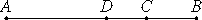# Proposition 42

A binomial straight line is divided into its terms at one point only.

Let AB be a binomial straight line divided into its terms at C. Then AC and CB are rational straight lines commensurable in square only.

I say that AB is not divided at another point into two rational straight lines commensurable in square only.

For, if possible, let it be divided at D also, so that AD and DB are also rational straight lines commensurable in square only.It is then manifest that AC is not the same as DB.

If possible, let it be so. Then AB is also the same as CB, and AC is to CB as BD is to DA. Thus AB is divided at D also in the same way as by the division at C, which is contrary to the hypothesis.

Therefore AC is not the same with DB.

For this reason also the points C and D are not equidistant from the point of bisection.

II.4

Therefore that by which the sum of the squares on AC and CB differs from the sum of the squares on AD and DB is also that by which twice the rectangle AD by DB differs from twice the rectangle AC by CB, because both the squares on AC and CB together with twice the rectangle AC by CB, and the squares on AD and DB together with twice the rectangle AD by DB, equal the square on AB.

But the sum of the squares on AC and CB differs from the sum of the squares on AD and DB by a rational area, for both are rational, therefore twice the rectangle AD by DB also differs from twice the rectangle AC by CB by a rational area, though they are medial, which is absurd, for a medial area does not exceed a medial by a rational area.

Therefore a binomial straight line is not divided at different points. Therefore it is divided at one point only.

Therefore, a binomial straight line is divided into its terms at one point only.

Q.E.D.

## Guide

This proposition is used in X.47.